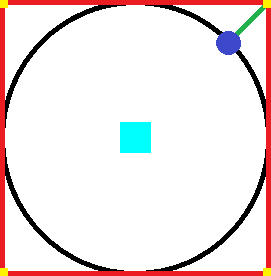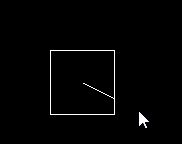# Mathematical thing (squared lengthdir)

Discussion in 'Programming' started by Edgamer63, Apr 21, 2019.

1. ### Edgamer63Member

Joined:
Jan 24, 2018
Posts:
66
Hello... well, what i'm trying to do is to limitate the x,y (even z) position of a vector... using a direction vector to get a 2D vector with angles like a circle... but it showing as a SquareWell... blue circle is how lengthdir works (circle)... but i want to get it working like the red square and having the yellow corners as a limit (also red square).

Sooo..... There's a way to do it?!

2. ### woodsMember

Joined:
Jun 21, 2016
Posts:
223
something like checking number of cells away not number of pixles?

Edgamer63 likes this.
3. ### Edgamer63Member

Joined:
Jan 24, 2018
Posts:
66
Probably... why not4. ### TheouAegisMember

Joined:
Jul 3, 2016
Posts:
6,653
x+lenthdir_x(big_radius, direction1)+lengthdir_x(small_radius,direction2)

?

That's assuming direction1 and direction2 are different.

Edgamer63 likes this.
5. ### jaydeeMember

Joined:
Dec 27, 2016
Posts:
75
You could use trigonometry:

In a right angled triangle, the tan of an angle is the length of the opposite line divided by the adjacent line:
tan(a) = Opposite / Adjacent.

https://www.mathsisfun.com/algebra/trig-four-quadrants.html

But since we need to vary between using the x axis distance, and the y axis distance depending on the angle, we will have to implement a system to check what quadrant we're using. Also remember that GMS uses an inverted y axis.

This is the sort of code you'll have to use (pseudo code, not gml):
Code:
```// Settings
var halfwidth = 32;
var halfheight = 32;
var originx = 0;
var originy = 0;

// Outputs
var outx, outy;

// I'm using radians, but you could use degrees as well

// Input angle must be between 0 and 2 * pi
angle = 3 * pi / 2;

if(angle >= 7 * pi / 4 || angle < pi / 4) {
outx = originx + halfwidth;
outy = originy - halfwidth * tan(angle); // Inverse y axis
}
else if(angle >= pi / 4 && angle < 3 * pi / 4) {
outx = originx - halfheight * tan(angle);
outy = originy - halfheight;
}
else if(angle >= 3 * pi / 4 && angle < 5 * pi / 4) {
outx = originx - halfwidth;
outy = originy + halfwidth * tan(angle);
}
else if(angle >= 5 * pi / 4 && angle < 7 * pi / 4) {
outx = originx + halfheight * tan(angle - 5 * pi / 4);
outy = originy + halfheight;
}```

Edgamer63 likes this.
6. ### MiradurMember

Joined:
Jan 16, 2018
Posts:
129
Why so complicated, just ask the border if your x and y are bigger. The commands are:

bbox_left()
bbox_right()
bbox_top()
bbox_bottom()

Miradur

7. ### TheSnidrHeavy metal viking dentistGMC Elder

Joined:
Jun 21, 2016
Posts:
462
Not tested, but I think this should work:
Code:
```var dx = dcos(angle);
var dy = - dsin(angle);
var m = length / max(abs(dx), abs(dy));
dx *= m;
dy *= m;```
It divides the coordinate by the largest of the x and y values, forcing it out to the square while still keeping the direction. Length is half the width and height of the square. Angle is in degrees.

Last edited: Apr 21, 2019
Edgamer63 and jaydee like this.
8. ### jaydeeMember

Joined:
Dec 27, 2016
Posts:
75
If they only want the x or y coordinate, but not both, then sure this works. But that wasn't the question as I interpreted it.

@TheSnidr Nice approach.

Edgamer63 and TheSnidr like this.
9. ### Edgamer63Member

Joined:
Jan 24, 2018
Posts:
66
Any of this solutions doesn't work properly... Also... the solution Provided by jaydee... wouldbe cool if worked with angles... but it didn't :'(

10. ### TheSnidrHeavy metal viking dentistGMC Elder

Joined:
Jun 21, 2016
Posts:
462
Then what exactly do you need?
I got around to testing my solution, and I'd say it works pretty well based on your question in the first post:Here's the code I used to draw this example:
Code:
```draw_rectangle(x - 32, y - 32, x + 32, y + 32, true);
var angle = point_direction(x, y, mouse_x, mouse_y);
var dx = dcos(angle);
var dy = - dsin(angle);
var m = 32 / max(abs(dx), abs(dy));
dx *= m;
dy *= m;
draw_line(x, y, x + dx, y + dy);```

Edgamer63 likes this.
11. ### Edgamer63Member

Joined:
Jan 24, 2018
Posts:
66
Thank You TheSnidr !